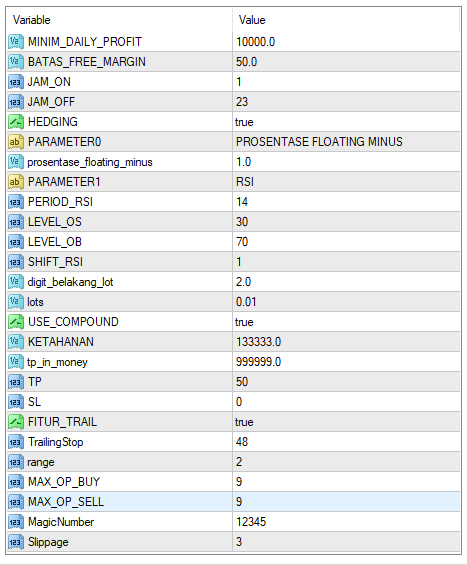If the initial deposit is \$1000 on MODERATE RISK micro account use the parameter settings below:

• Currency pairs: EUR/USD
• Timeframe: H1
• Leverage: 1:500
• Swap free account (forex robot will hold floating loss for weeks or even months)
Parameter settings for EUR/USD, H1
• MINIM_DAILY_PROFIT Minimum daily profit = 10000
• BATAS_FREE_MARGIN Free margin limit = 50
• JAM_ON The robot starts trading = 1
• JAM_OFF = The robot is finished trading = 23
• HEDGING Hedging = true
• PARAMETER0 = PROSENTASE FLOATING MINUS
• prosentase_floating_minus = Hedging based on a percentage = 1
• PARAMETER1 = Indicators used = RSI
• PERIOD_RSI Period RSI = 14
• LEVEL_OS = Level OS = 30
• LEVEL_OB Level OB = 70
• SHIFT_RSI Shift = 1
• digit_belakang_lot Back digit lot= 2
• lots = Lot = 0.01
• USE_COMPOUND Using compounding = true
• KETAHANAN Endurance = 133333
• tp_in_money Take profit in money = 999999
• TP = take profit = 50
• SL = Stop loss = 0
• FITUR_TRAIL = Trailingstop feature = true
• TrailingStop = Trailingstop = 48
• range = Range = 2
• MAX_OP_SELL = Maximum open sell = 9
• MagicNumber = Magicnumber = 12345
• Slippage = Slippage = 3